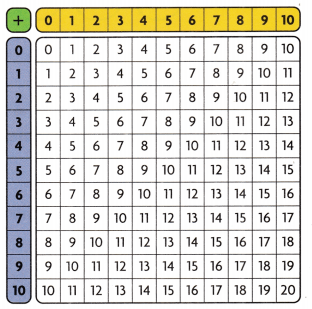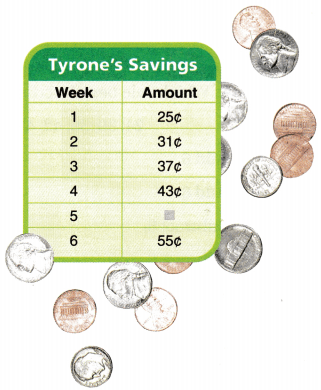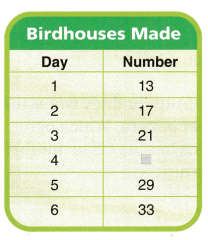# Texas Go Math Grade 3 Lesson 14.3 Answer Key Number Patterns

Refer to our Texas Go Math Grade 3 Answer Key Pdf to score good marks in the exams. Test yourself by practicing the problems from Texas Go Math Grade 3 Lesson 14.3 Answer Key Number Patterns.

## Texas Go Math Grade 3 Lesson 14.3 Answer Key Number Patterns

Unlock the Problem

A pattern is an ordered set of numbers or objects. The order helps you predict what will come next.You can use the addition table to explore patterns.

Activity 1

Materials; orange and green crayons
Look across each row and down each column. What pattern do you see?

Shade the row and column orange for the addend 0. Compare the shaded squares to the yellow row and the blue column. What pattern do you see?

What happens when you add 0 to a number?

Shade the row and column green for the addend 1. What pattern do you see?

What happens when you add 1 to a number?
Answer: The number will increase by 1

Math Talk
Mathematical Processes

What other patterns can you find in the addition table?

More Number Patterns

A rule can be used to describe a pattern.
Math Idea
A rule must be true for the whole pattern.

Look at this number pattern. Find a rule.
Think: What do I do to 1 to get 4? What do I do to 4 to get 7?Explanation:
1+3=4, 4+3=7, 7+3=10, 10+3=13. So updated the number to fine rule
What is a rule for this pattern?
Explanation:
1+3=4, 4+3=7, 7+3=10, 10+3=13. So pattern is Add3.

Use the rule above to write the next three numbers.
1, 4, 7, 10, 13, _____, ______, ______
Answer: next three numbers are 16, 19, 22
Explanation:
1+3=4, 4+3=7, 7+3=10, 10+3=13, 13+3=16, 16+3=19, 19+3=22

Use the rule above to find the missing number.
2, 5, _____, 11, 14
Explanation:
Add 3 rule , which gives missing number is 8

Use the rule Subtract 3 to create a pattern. Write the first five numbers in the pattern.
Think: What number should I start with? What numbers do I get when I subtract 3?
Explanation:
13-10=3, 10-3=7, 7-3=4, 4-3=1

Share and Show

Write a rule for the pattern. Then write the next two numbers.

Question 1.
21, 26, 31, 36, _____, ______
Rule: ______________
Next two numbers are 41,46
Explanation:
21+5=26, 26+5=31, 31+5=36,  36+5=41, 41+5=46

Write a rule for the pattern. Then write the missing number.

Question 2.
38, 35, _____, 29, 26, 23, 20
Rule: _________________
Missing Number is 32
Explanation:
38-3=35, 35-3=32, 32-3=29, 29-3=26, 26-3=23, 23-3=20

Use the rule to create a pattern. Write the first five numbers in the pattern.

Go Math Grade 3 Number Patterns Lesson 14.3 Answer Key Question 3.
Answer: 1, 5, 9, 13, 17
Explanation:
1+4=5, 5+4=9, 9+4=13, 13+4=17

Problem Solving

Use the table for 4 – 6.Question 4.
How much money does Tyrone save each week after Week 1?
Explanation:
25+6=31, 31+6=37, 37+6=43,43+6=49, 49+6=55
Question 5.
How much money has Tyrone saved by the end of Week 5?
Explanation:
25+6=31, 31+6=37, 37+6=43,43+6=49, 49+6=55

Question 6.
Write Math Tyrone wants to buy a book that costs 75C. If he continues his pattern of saving, will he have enough saved by Week 10? Explain.
Explanation: By end of 10th week, he can save 79C, if he continue his pattern of saving. Since
25+6=31, 31+6=37, 37+6=43,43+6=49, 49+6=55, 55+6=61, 61+6=67, 67+6=73, 73+6=79.
So he has enough money to buy a book.

Question 7.
H.O.T. Use Math Language Diego created the pattern below. Write a rule for the pattern. Then write the missing number.
42, 43, 45, 48, 52, __________Answer: 42, 43, 45, 48, 52, 57
Explanation:
The difference will increase by adding 1
The difference between 42 and 43 is 1
The difference between 43 and 45 is 2
The difference between 45 and 48 is 3
The difference between 48 and 52 is 4
The difference between 52 and 57 is 5

3rd Grade Go Math Lesson 14.3 Number Patterns Question 8.
H.O.T. Multi-Step Create your own pattern by starting with a 2-digit number. Write a rule for the pattern so that a 1-digit number is added to find the next number. Write the first five numbers in your pattern.
Answer: 23, 25, 27, 29, 31
Explanation: Pattern started with 2 digit number as 23. Rule is ADD 2. So first five numbers are
23+2=25, 25+2=27, 27+2=29, 29+2=31

Fill in the bubble for the correct answer choice.

Question 9.
Analyze Shelly creates the number pattern shown below. Which rule best describes her pattern?
24, 30, 36, 42, 48, _________
(C) Subtract 6.
(D) Subtract 8.
Explanation:
Add 6 is the pattern, Since 24+6=30, 30+6=36, 36+6=42, 42+6=48

Question 10.
What is the next number in the pattern shown below?
58, 54, 50, 46, 42, __________
(A) 44
(B) 36
(C) 38
(D) 41
Explanation:
Next number is 38. Since 58-4=54, 54-4=50, 50-4=46, 46-4=42, 42-4=38

Question 11.
Multi-Step Two patterns are shown below. Jerry wrote a third pattern. The first number of his pattern is the sum of the missing numbers below. What is the first number of the third pattern?
12, 17, _____, 27, 32
34, 30, 26, _____, 18
(A) 5
(B) 22
(C) 4
(D) 44
Explanation:
12, 17, _____, 27, 32  — Rule is Add 5 and Missing Number is 22.
34, 30, 26, _____, 18 — Rule is Subtract 4 and Missing number is 22
So Sum of missing numbers are 22 +22= 44.
So The first number of his pattern is 44.

Texas Test Prep

Which rule best describes the pattern?
85, 80, 75, 70, 65, 60, 55, 50
(B) Subtract 5.
(C) Subtract 10.
Explanation:
85-5=80, 80-5=75, 75-5=70, 70-5=65, 65-5=60, 60-5=55, 55-5=50

### Texas Go Math Grade 3 Lesson 14.3 Homework and Practice Answer Key

Write the rule for the pattern. Then write the next two numbers.

Question 1.
23, 26, 29, 32, _____, ____
Rule: ___________
Answer: Rule is Add 3 and Next two numbers are 35, 38
Explanation:
23+3=26, 26+3=29, 29+3=32, 32+3=35, 35+3=38

Question 2.
48, 44, 40, 36, _____, _____
Rule: ___________
Answer: Rule is Subtract 4 and Next two numbers are 32, 28
Explanation:
48-4=44, 44-4=40, 40-4=36, 36-4=32, 32-4=28

Use the rule to create a pattern. Write the first five numbers in the pattern.

Question 3.
Subtract 5.
Answer: 39, 34, 29, 24, 19, 14
Explanation:
Subtract 5 number pattern 39, 34, 29, 24, 19, 14 is 39-5=34, 34-5=29, 29-5=24, 24-5=19, 19-5=14
Question 4.
Answer: 10, 16, 22, 28, 34, 40
Explanation:
Add 6 is the pattern, Since 24+6=30, 30+6=36, 36+6=42, 42+6=48

Problem Solving

Use the table for 5-7.Question 5.
A craft club is making birdhouses to sell for charity. How many birdhouses do they make at the end of day 4?
Explanation:
They are selling 4 birdhouses each day. we can find based on pattern. So they sell 25 end of 4 day. Since
13+4=17, 17+4=21, 21+4=25

Question 6.
What is the rule for the pattern of making birdhouses?
Explanation:
They are selling 4 birdhouses each day. we can find based on pattern.  Since
13+4=17, 17+4=21, 21+4=25

Question 7.
The club wants to make a total of 45 birdhouses. If they continue the pattern of making birdhouses, on which day will they have 45 birdhouses?
Explanation:
They are selling 4 birdhouses each day. we can find based on pattern. Since
13+4=17, 17+4=21, 21+4=25, 25+4=29, 29+4=33, 33+4=37, 37+4=41, 41+4=45

Question 8.
Nadia created the pattern below. Write a rule for the pattern. Then write the missing number.
10, 11, 9, 10, 8, 9, _________
Rule is increase by 1 and decrease by 2
Explanation:
10, 11, 9, 10, 8, 9 decrease by 2 that is 7

Lesson Check

Question 9.
Axle creates this number pattern. Which rule best describes his pattern?
39, 34, 29, 24, 19, _______
(A) Subtract 4.
(C) Subtract 5.
Explanation:
Axle created Subtract 5 number pattern because 39-5=34, 34-5=29, 29-5=24, 24-5=19, 19-5=14
So next number in the pattern is 14.
39, 34, 29, 24, 19, 14

Question 10.
Latisha creates this number pattern. Which rule best describes her pattern?
5, 12, 19, 26, 33, ________
(C) Subtract 6.
(D) Subtract 7.
Explanation:
Latisha created Add 7 number pattern. Since
5+7=12, 12+7=19, 19+7=26, 26+7=33, 33+6=39. So next number in the pattern is
5, 12, 19, 26, 33, __39__

Darla writes the number pattern shown below. What is the next number?
53, 50, 47, 44, 41, ________
(A) 42
(B) 40
(C) 39
(D) 38
Explanation:
53, 50, 47, 44, 41, ________ Next Number is 38. Since
53-3=50, 50-3=47, 47-3=44, 44-3=41, 41-3=38

Question 12.
Soo Jin writes the number pattern shown below. What is the next number?
54, 60, 66, 72, 78, _______
(A) 85
(B) 80
(C) 79
(D) 84
Explanation:
54, 60, 66, 72, 78, _______ Next number is 84. Since
54+6=60, 60+6=66, 66+6=72, 72+6=78, 78+6=84

Question 13.
Multi-Step Rodney writes this number pattern. Which of the patterns has the same number that comes next?
13, 16, 19, 22, ________
(A) 5, 10, 15, 20, ________
(B) 16, 18, 20, 22, ________
(C) 6, 12, 18, 24, ________
(D) 12, 15, 18, 21, ________
Answer: (A) 5, 10, 15, 20, ________
Explanation:
In Rodney written pattern 13, 16, 19, 22, ___25_____ next number is 25. Since
13+3=16, 16+3=19, 19+3=22, 22+3=25.
Then option A is the answer because same number comes next in that pattern.
5, 10, 15, 20, ___25_ Since
5+5=10, 10+5=15, 15+5=20, 20+5=25

Question 14.
Multi-Step The missing numbers in these two patterns add up to a secret number. What is the secret number?
15, 21, ________, 33, 39
19, ________, 27, 31, 35
(A) 27
(B) 23
(C) 40
(D) 50